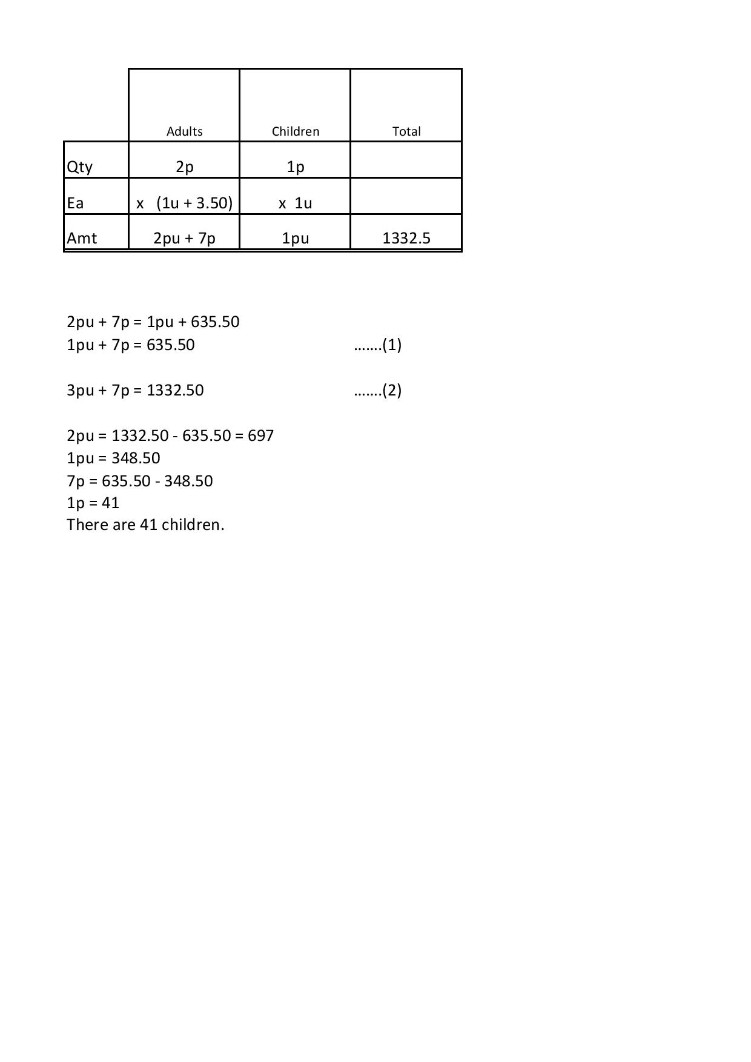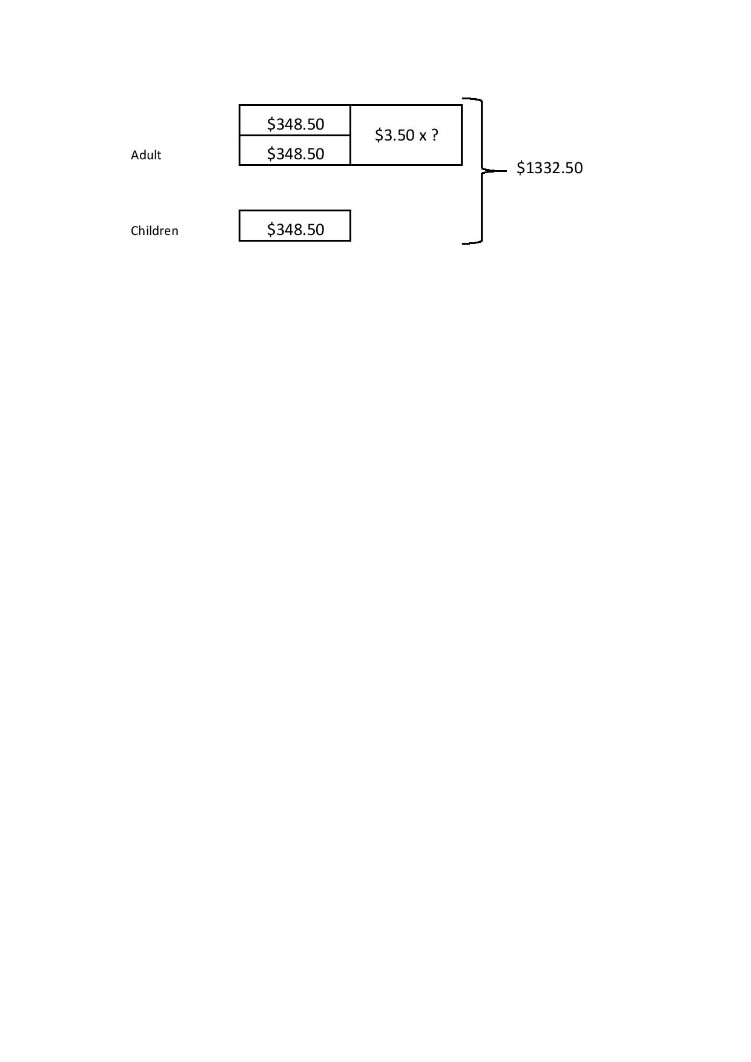# AnswerThe above is one way to solve this question. If you don’t like it, here is another way.

Find the money collected from sales of adult tickets and sales of children’s tickets.

Total collected = \$1332.50

Amount collected from adult tickets = (\$1332.50 + \$635.50) ÷ 2 = \$984

Amount collected from children’s tickets = \$1332.50 – \$984 = \$348.50

Since there are twice as many adults as children, multiply \$348.50 by 2.

348.50 × 2 = 697

984 – 697 = 287

The \$287 must be accounted for by the extra cost of an adult ticket (\$3.50) over a child ticket.

287 ÷ 3.50 = 82

There are 82 adults and 41 children.

2 Replies 0 Likes ✔Accepted AnswerHere is a model representation of the last part to find the number of adults and children.

0 Replies 0 Likes